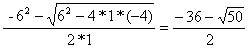### (1) is a correct answer.

Because:=>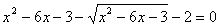Let z =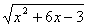.  The rewrite equation as z2 - z -2 = 0,

Factor (z-2)(z-1) = 0 or z = 2, z = 1.

For z = 2=or x2 + 6x -3 = 4 => x2 + 6x - 7 = 0 => (x+7)(x-1) = 0 => x=-7 or x=-1

A second correct answer would be

For z = 1=or x2 + 6x -3 = 1 => x2 + 6x - 4 = 0 use quadratic formula to solve ax2 + bx +c = 0. x =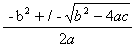x=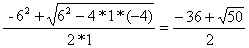or x=